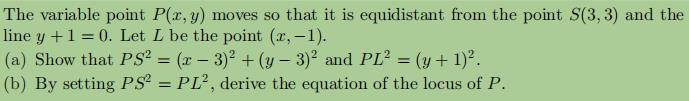### Still have math questions?

Algebra
QuestionThe variable point $$P ( x , y )$$ moves so that it is equidistant from the point $$S ( 3,3 )$$ and the line $$y + 1 = 0$$ . Let $$L$$ be the point $$( x , - 1 )$$ .

(a) Show that $$P S ^ { 2 } = ( x - 3 ) ^ { 2 } + ( y - 3 ) ^ { 2 }$$ and $$P L ^ { 2 } = ( y + 1 ) ^ { 2 }$$ .

(b) By setting $$P S ^ { 2 } = P L ^ { 2 }$$ , derive the equation of the locus of $$P$$ .

$$\text { (b) } ( x - 3 ) ^ { 2 } = 8 ( y - 1 )$$